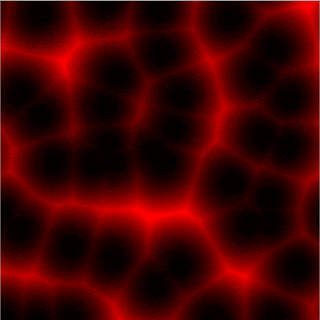### Noise Generation Algorithms : Voronoi Part 2

If you haven't gone through Part 1, do so now or perish.😁.
Now we will go over making a more cooler version of what we previously did.
This is what we will end up with:Voronoi Noise Shader In Unity
In this part we will go over the actual 'Noise' part of it.
For that we need a pseudo random number generator, We already made one in a previous tutorial on making White Noise with shaders.
But for this case we need to make 2D noise rather than 1D noise like we did before.
So we will go over our random function:
``````float2 random2(float2 p)
{
return frac(sin(float2(dot(p,float2(117.12,341.7)),dot(p,float2(269.5,123.3))))*43458.5453);
}
``````
If you have gone the previous pseudo-random number generator that was made.. nothing really complicated is happening here.
We are still using the 'frac' function to give us the fractional part of a a number made with the 'dot' function which contains a float2 and some arbitrary numbers as parameters.
Now the fragment shader where all the magic happens:
``````fixed4 frag(v2f i) : SV_Target
{
fixed4 col = fixed4(0,0,0,1);
float2 uv = i.uv;
uv *= 6.0; //Scaling amount (larger number more cells can be seen)
float2 iuv = floor(i.uv); //gets integer values no floating point
float2 fuv = frac(i.uv); // gets only the fractional part
float minDist = 1.0;  // minimun distance
for (int y = -1; y <= 1; y++)
{
for (int x = -1; x <= 1; x++)
{
// Position of neighbour on the grid
float2 neighbour = float2(float(x), float(y));
// Random position from current + neighbour place in the grid
float2 pointv = random2(iuv + neighbour);
// Move the point with time
pointv = 0.5 + 0.5*sin(_Time.z + 6.2236*pointv);//each point moves in a certain way
// Vector between the pixel and the point
float2 diff = neighbour + pointv - fuv;
// Distance to the point
float dist = length(diff);
// Keep the closer distance
minDist = min(minDist, dist);
}
}
// Draw the min distance (distance field)
col.r += minDist * minDist; // squared it to to make edges look sharper
return col;
}
``````
Play around with different values and see what comes up.
The shader source code can be found HERE.
If you like programming shaders make sure you check these out : Shader Tutorials
Support Bitshift Programmer by leaving a like on Bitshift Programmer Facebook Page and be updated as soon as there is a new blog post.